Digital video plays a central role in today’s communication, information consumption, entertainment and educational approaches, and has enormous economic and sociocultural impacts on everyday life. In the first decade of the 21st century, the profound dominance of video as an information medium on modern life—from digital television to Skype, DVD to Blu-ray, and YouTube to Netflix–has been well established. Owing to the enormous amount of data required to represent digital video, it is necessary to compress the video data for practical transmission and communication, storage, and streaming applications.

In this chapter we start with a brief discussion of the limits of digital networks and the extent of compression required for digital video transmission. This sets the stage for further discussions on compression. It is followed by a discussion of the human visual system (HVS) and the compression opportunities allowed by the HVS. Then we explain the terminologies, data structures, and concepts commonly used in digital video compression.

We discuss various redundancy reduction and entropy coding techniques that form the core of the compression methods. This is followed by overviews of various compression techniques and their respective advantages and limitations. We briefly introduce the rate-distortion curve both as the measure of compression efficiency and as a way to compare two encoding solutions. Finally, there’s a discussion of the factors influencing and characterizing the compression algorithms before a brief summary concludes the chapter.

## Network Limits and Compression

Before the advent of the Integrated Services Digital Network (ISDN), the Plain Old Telephone Service (POTS)was the commonly available network, primarily to be used for voice-grade telephone services based on analog signal transmission. However, the ubiquity of the telephone networks meant that the design of new and innovative communication services such as facsimile (fax) and modem were initially inclined toward using these available analog networks. The introduction of ISDN enabled both voice and video communication to engage digital networks as well, but the standardization delay in Broadband ISDN (B-ISDN) allowed packet-based local area networks such as the Ethernet to become more popular. Today, a number of network protocols support transmission of images or videos using wire line or wireless technologies, having different bandwidth and data-rate capabilities, as listed in Table 2-1.

In the 1990s, transmission of raw digital video data over POTS or ISDN was unproductive and very expensive due to the sheer data rate required. Note that the raw data rate for the ITU-R 601 formatsFootnote 1 is ∼165 Mbps (million bits per second), beyond the networks’ capabilities. In order to partially address the data-rate issue, the 15th specialist group (SGXV) of the CCITT Footnote 2 defined the Common Image Format (CIF)to have common picture parameter values independent of the picture rate. While the format specifies many picture rates (24 Hz, 25 Hz, 30 Hz, 50 Hz, and 60 Hz), with a resolution of 352 × 288 at 30 Hz, the required data rate was brought down to approximately 37 Mbps, which would typically fit into a basic Digital Signal 0 (DS0) circuit, and would be practical for transmission.

With increased compute capabilities, video encoding and processing operations became more manageable over the years. These capabilities fueled the growing demand of ever higher video resolutions and data rates to accommodate diverse video applications with better-quality goals. One after another, the ITU-R Recommendations BT.601,Footnote 3 BT.709,Footnote 4 and BT.2020Footnote 5 appeared to support video formats with increasingly higher resolutions. Over the years these recommendations evolved. For example, the recommendation BT.709, aimed at high-definition television (HDTV), started with defining parameters for the early days of analog high-definition television implementation, as captured in Part 1 of the specification. However, these parameters are no longer in use, so Part 2 of the specification contains HDTV system parameters with square pixel common image format.

Meanwhile, the network capabilities also grew, making it possible to address the needs of today’s industries. Additionally, compression methods and techniques became more refined.

## The Human Visual System

The human visual system (HVS) is part of the human nervous system, which is managed by the brain. The electrochemical communication between the nervous system and the brain is carried out by about 100 billion nerve cells, called neurons. Neurons either generate pulses or inhibit existing pulses, and result in a variety of phenomena ranging from Mach bands, band-pass characteristic of the visual frequency response, to the edge-detection mechanism of the eye. Study of the enormously complex nervous system is manageable because there are only two types of signals in the nervous system: one for long distances and the other for short distances. These signals are the same for all neurons, regardless of the information they carry, whether visual, audible, tactile, or other.

Understanding how the HVS works is important for the following reasons:

• It explains how accurately a viewer perceives what is being presented for viewing.

• It helps understand the composition of visual signals in terms of their physical quantities, such as luminance and spatial frequencies, and helps develop measures of signal fidelity.

• It helps represent the perceived information by various attributes, such as brightness, color, contrast, motion, edges, and shapes. It also helps determine the sensitivity of the HVS to these attributes.

• It helps exploit the apparent imperfection of the HVS to give an impression of faithful perception of the object being viewed. An example of such exploitation is color television. When it was discovered that the HVS is less sensitive to loss of color information, it became easy to reduce the transmission bandwidth of color television by chroma subsampling.

The major components of the HVS include the eye, the visual pathways to the brain, and part of the brain called the visual cortex. The eye captures light and converts it to signals understandable by the nervous system. These signals are then transmitted and processed along the visual pathways.

So, the eye is the sensor of visual signals. It is an optical system, where an image of the outside world is projected onto the retina, located at the back of the eye. Light entering the retina goes through several layers of neurons until it reaches the light-sensitive photoreceptors, which are specialized neurons that convert incident light energy into neural signals.

There are two types of photoreceptors: rods and cones. Rods are sensitive to low light levels; they are unable to distinguish color and are predominant in the periphery. They are also responsible for peripheral vision and they help in motion and shape detection. As signals from many rods converge onto a single neuron, sensitivity at the periphery is high, but the resolution is low. Cones, on the other hand, are sensitive to higher light levels of long, medium, and short wavelengths. They form the basis of color perception. Cone cells are mostly concentrated in the center region of the retina, called the fovea. They are responsible for central or foveal vision, which is relatively weak in the dark. Several neurons encode the signal from each cone, resulting in high resolution but low sensitivity.

The number of the rods, about 100 million, is higher by more than an order of magnitude compared to the number of cones, which is about 6.5 million. As a result, the HVS is more sensitive to motion and structure, but it is less sensitive to loss in color information. Furthermore, motion sensitivity is stronger than texture sensitivity; for example, a camouflaged still animal is difficult to perceive compared to a moving one. However, texture sensitivity is stronger than disparity; for example, 3D depth resolution does not need to be so accurate for perception.

Even if the retina perfectly detects light, that capacity may not be fully utilized or the brain may not be consciously aware of such detection, as the visual signal is carried by the optic nerves from the retina to various processing centers in the brain. The visual cortex, located in the back of the cerebral hemispheres, is responsible for all high-level aspects of vision.

Apart from the primary visual cortex, which makes up the largest part of the HVS, the visual signal reaches to about 20 other cortical areas, but not much is known about their functions. Different cells in the visual cortex have different specializations, and they are sensitive to different stimuli, such as particular colors, orientations of patterns, frequencies, velocities, and so on.

Simple cells behave in a predictable fashion in response to particular spatial frequency, orientation, and phase, and serve as an oriented band-pass filter. Complex cells, the most common cells in the primary visual cortex, are also orientation-selective, but unlike simple cells, they can respond to a properly oriented stimulus anywhere in their receptive field. Some complex cells are direction-selective and some are sensitive to certain sizes, corners, curvatures, or sudden breaks in lines.

The HVS is capable of adapting to a broad range of light intensities or luminance, allowing us to differentiate luminance variations relative to surrounding luminance at almost any light level. The actual luminance of an object does not depend on the luminance of the surrounding objects. However, the perceived luminance, or the brightness of an object, depends on the surrounding luminance. Therefore, two objects with the same luminance may have different perceived brightnesses in different surroundings. Contrast is the measure of such relative luminance variation. Equal logarithmic increments in luminance are perceived as equal differences in contrast. The HVS can detect contrast changes as low as 1 percent.Footnote 6

### The HVS Models

The fact that visual perception employs more than 80 percent of the neurons in human brain points to the enormous complexity of this process. Despite numerous research efforts in this area, the entire process is not well understood. Models of the HVS are generally used to simplify the complex biological processes entailing visualization and perception. As the HVS is composed of nonlinear spatial frequency channels, it can be modeled using nonlinear models. For easier analysis, one approach is to develop a linear model as a first approximation, ignoring the nonlinearities. This approximate model is then refined and extended to include the nonlinearities. The characteristics of such an example HVS modelFootnote 7 include the following.

#### The First Approximation Model

This model considers the HVS to be linear, isotropic, and time- and space-invariant. The linearity means that if the intensity of the light radiated from an object is increased, the magnitude of the response of the HVS should increase proportionally. Isotropic implies invariance to direction. Although, in practice, the HVS is anisotropic and its response to a rotated contrast grating depends on the frequency of the grating, as well as the angle of orientation, the simplified model ignores this nonlinearity. The spatio-temporal invariance is difficult to modify, as the HVS is not homogeneous. However, the spatial invariance assumption partially holds near the optic axis and the foveal region. Temporal responses are complex and are not generally considered in simple models.

In the first approximation model, the contrast sensitivity as a function of spatial frequency represents the optical transfer function (OTF) of the HVS. The magnitude of the OTF is called the modulation transfer function (MTF), as shown in Figure 2-1.

The curve representing the thresholds of visibility at various spatial frequencies has an inverted U-shape, while its magnitude varies with the viewing distance and viewing angle. The shape of the curve suggests that the HVS is most sensitive to mid-frequencies and less sensitive to high frequencies, showing band-pass characteristics.

The MTF can thus be represented by a band-pass filter. It can be modeled more accurately as a combination of a low-pass and a high-pass filter. The low-pass filter corresponds to the optics of the eye. The lens of the eye is not perfect, even for persons with no weakness of vision. This imperfection results in spherical aberration, appearing as a blur in the focal plane. Such blur can be modeled as a two-dimensional low-pass filter. The pupil’s diameter varies between 2 and 9 mm. This aperture can also be modeled as a low-pass filter with high cut-off frequency corresponding to 2 mm, while the frequency decreases with the enlargement of the pupil’s diameter.

On the other hand, the high-pass filter accounts for the following phenomenon. The post-retinal neural signal at a given location may be inhibited by some of the laterally located photoreceptors. This is known as lateral inhibition, which leads to the Mach band effect, where visible bands appear near the transition regions of a smooth ramp of light intensity. This is a high-frequency change from one region of constant luminance to another, and is modeled by the high-pass portion of the filter.

#### Refined Model Including Nonlinearity

The linear model has the advantage that, by using the Fourier transform techniques for analysis, the system response can be determined for any input stimulus as long as the MTF is known. However, the linear model is insufficient for the HVS as it ignores important nonlinearities in the system. For example, it is known that light stimulating the receptor causes a potential difference across the membrane of a receptor cell, and this potential mediates the frequency of nerve impulses. It has also been determined that this frequency is a logarithmic function of light intensity (Weber-Fechner law). Such logarithmic function can approximate the nonlinearity of the HVS. However, some experimental results indicate a nonlinear distortion of signals at high, but not low, spatial frequencies.

These results are inconsistent with a model where logarithmic nonlinearity is followed by linear independent frequency channels. Therefore, the model most consistent with the HVS is the one that simply places the low-pass filter in front of the logarithmic nonlinearity, as shown in Figure 2-2. This model can also be extended for spatial vision of color, in which a transformation from spectral energy space to tri-stimulus space is added between the low-pass filter and the logarithmic function, and the low-pass filter is replaced with three independent filters, one for each band.

#### The Model Implications

The low-pass, nonlinearity, high-pass structure is not limited to spatial response, or even to spectral-spatial response. It was also found that this basic structure is valid for modeling the temporal response of the HVS. A fundamental premise of this model is that the HVS uses low spatial frequencies as features. As a result of the low-pass filter, rapid discrete changes appear as continuous changes. This is consistent with the appearance of discrete time-varying video frames as continuous-time video to give the perception of smooth motion.

This model also suggests that the HVS is analogous to a variable bandwidth filter, which is controlled by the contrast of the input image. As input contrast increases, the bandwidth of the system decreases. Therefore, limiting the bandwidth is desirable to maximize the signal-to-noise ratio. Since noise typically contains high spatial frequencies, it is reasonable to limit this end of the system transfer function. However, in practical video signals, high-frequency details are also very important. Therefore, with this model, noise filtering can only be achieved at the expense of blurring the high-frequency details, and an appropriate tradeoff is necessary to obtain optimum system response.

#### The Model Applications

In image recognition systems, a correlation may be performed between low spatial-frequency filtered images and stored prototypes of the primary receptive area for vision, where this model can act as a pre-processor. For example, in recognition and analysis of complex scenes with variable contrast information, when a human observer directs his attention to various subsections of the complex scene, an automated system based on this model could compute average local contrast of the subsection and adjust filter parameters accordingly. Furthermore, in case of image and video coding, this model can also act as a pre-processor to appropriately reflect the noise-filtering effects, prior to coding only the relevant information. Similarly, it can also be used for bandwidth reduction and efficient storage systems as pre-processors.

A block diagram of the HVS model is shown in Figure 2-3, where parts related to the lens, the retina, and the visual cortex, are indicated.

In Figure 2-3, the first block is a spatial, isotropic, low-pass filter. It represents the spherical aberration of the lens, the effect of the pupil, and the frequency limitation by the finite number of photoreceptors. It is followed by the nonlinear characteristic of the photoreceptors, represented by a logarithmic curve. At the level of the retina, this nonlinear transformation is followed by an isotropic high-pass filter corresponding to the lateral inhibition phenomenon. Finally, there is a directional filter bank that represents the processing performed by the cells of the visual cortex. The bars in the boxes indicate the directional filters. This is followed by another filter bank, represented by the double waves, for detecting the intensity of the stimulus. It is worth mentioning that the overall system is shift-variant because of the decrease in resolution away from the fovea.Footnote 8

### Expoliting the HVS

By taking advantage of the characteristics of the HVS, and by tuning the parameters of the HVS model, tradeoffs can be made between visual quality loss and video data compression. In particular, the following benefits may be accrued.

• By limiting the bandwidth, the visual signal may be sampled in spatial or temporal dimensions at a frequency equal to twice the bandwidth, satisfying the Nyquist criteria of sampling, without loss of visual quality.

• The sensitivity of the HVS is decreased during rapid large-scale scene change and intense motion of objects, resulting in temporal or motion masking. In such cases the visibility thresholds are elevated due to temporal discontinuities in intensity. This can be exploited to achieve more efficient compression, without producing noticeable artifacts.

• Texture information can be compressed more than motion information with negligible loss of visual quality. As discussed later in this chapter, several lossy compression algorithms allow quantization and resulting quality loss of texture information, while encoding the motion information losslessly.

• Owing to low sensitivity of the HVS to the loss of color information, chroma subsampling is a feasible technique to reduce data rate without significantly impacting the visual quality.

• Compression of brightness and contrast information can be achieved by discarding high-frequency information. This would impair the visual quality and introduce artifacts, but parameters of the amount of loss are controllable.

• The HVS is sensitive to structural distortion. Therefore, measuring such distortions, especially for highly structured data such as image or video, would give a criterion to assess whether the amount of distortion is acceptable to human viewers. Although acceptability is subjective and not universal, structural distortion metrics can be used as an objective evaluation criterion.

• The HVS allows humans to pay more attention to interesting parts of a complex image and less attention to other parts. Therefore, it is possible to apply different amount of compression on different parts of an image, thereby achieving a higher overall compression ratio. For example, more bits can be spent on the foreground objects of an image compared to the background, without substantial quality impact.

## An Overview of Compression Techniques

A high-definition uncompressed video data stream requires about 2 billion bits per second of data bandwidth. Owing to the large amount of data necessary to represent digital video, it is desirable that such video signals are easy to compress and decompress, to allow practical storage or transmission. The term data compression refers to the reduction in the number of bits required to store or convey data—including numeric, text, audio, speech, image, and video—by exploiting statistical properties of the data. Fortunately, video data is highly compressible owing to its strong vertical, horizontal, and temporal correlation and its redundancy.

Transform and prediction techniques can effectively exploit the available correlation, and information coding techniques can take advantage of the statistical structures present in video data. These techniques can be lossless, so that the reverse operation (decompression) reproduces an exact replica of the input. In addition, however, lossy techniques are commonly used in video data compression, exploiting the characteristics of the HVS, which is less sensitive to some color losses and some special types of noises.

Video compression and decompression are also known as video encoding and decoding, respectively, as information coding principles are used in the compression and decompression processes, and the compressed data is presented in a coded bit stream format.

### Data Structures and Concepts

Digital video signal is generally characterized as a form of computer data. Sensors of video signals usually output three color signals–red, green and blue (RGB)—that are individually converted to digital forms and are stored as arrays of picture elements (pixels), without the need of the blanking or sync pulses that were necessary for analog video signals. A two-dimensional array of these pixels, distributed horizontally and vertically, is called an image or a bitmap, and represents a frame of video. A time-dependent collection of frames represents the full video signal. There are five parametersFootnote 9 associated with a bitmap: the starting address in memory, the number of pixels per line, the pitch value, the number of lines per frame, and the number of bits per pixel. In the following discussion, the terms frame and image are used interchangeably.

#### Signals and Sampling

The conversion of a continuous analog signal to a discrete digital signal, commonly known as the analog-to-digital (A/D) conversion, is done by taking samples of the analog signal at appropriate intervals in a process known as sampling. Thus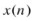is called the sampled version of the analog signal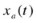if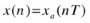for some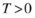, where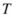is known as the sampling period and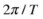is known as the sampling frequency or the sampling rate. Figure 2-4 shows a spatial domain representation of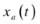and corresponding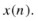The frequency-domain representation of the signal is obtained by using the Fourier transform, which gives the analog frequency response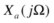replicated at uniform intervals, while the amplitudes are reduced by a factor of. Figure 2-5 shows the concept.

If there is overlap between the shifted versions of, aliasing occurs because there are remnants of the neighboring copies in an extracted signal. However, when there is no aliasing, the signalcan be recovered from its sampled versionby retaining only one copy.Footnote 10 Thus if the signal is band-limited within a frequency band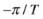to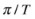, a sampling rate ofor more guarantees an alias-free sampled signal, where no actual information is lost due to sampling. This is called the Nyquist sampling rate, named after Harry Nyquist, who in 1928 proposed the above sampling theorem. Claude Shannon proved this theorem in 1949, so it is also popularly known as Nyquist-Shannon sampling theorem.

The theorem applies to single- and multi-dimensional signals. Obviously, compression of the signal can be achieved by using fewer samples, but in the case of sampling frequency less than twice the bandwidth of the signal, annoying aliasing artifacts will be visible.

#### Common Terms and Notions

There are a few terms to know that are frequently used in digital video. The aspect ratio of a geometric shape is the ratio between its sizes in different dimensions. For example, the aspect ratio of an image is defined as the ratio of its width to its height. The display aspect ratio (DAR) is the width to height ratio of computer displays, where common ratios are 4:3 and 16:9 (widescreen). An aspect ratio for the pixels within an image is also defined. The most commonly used pixel aspect ratio (PAR) is 1:1 (square); other ratios, such as 12:11 or 16:11, are no longer popular. The term storage aspect ratio (SAR) is used to describe the relationship between the DAR and the PAR such that SAR × PAR = DAR.

Historically, the role of pixel aspect ratio in the video industry has been very important. As digital display technology, digital broadcast technology, and digital video compression technology evolved, using the pixel aspect ratio has been the most popular way to address the resulting video frame differences. However, today, all three technologies use square pixels predominantly.

As other colors can be obtained from a linear combination of primary colors such as red, green and blue in RGB color model, or cyan, magenta, yellow, and black in CMYK model, these colors represent the basic components of a color space spanning all colors. A complete subset of colors within a given color space is called a color gamut. Standard RGB (sRGB) is the most frequently used color space for computers. International Telecommunications Union (ITU) has recommended color primaries for standard definition (SD), high-definition (HD) and ultra-high-definition (UHD) televisions. These recommendations are included in internationally recognized digital studio standards defined by ITU-R recommendation BT.601,Footnote 11 BT.709, and BT.2020, respectively. The sRGB uses the ITU-R BT.709 color primaries.

Luma is the brightness of an image, and is also known as the black-and-white information of the image. Although there are subtle differences between luminance as used in color science and luma as used in video engineering, often in the video discussions these terms are used interchangeably. In fact, luminance refers to a linear combination of red, green, and blue color representing the intensity or power emitted per unit area of light, while luma refers to a nonlinear combination of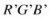, the nonlinear function being known as the gamma function (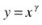, γ = 0.45). The primes are used to indicate nonlinearity. The gamma function is needed to compensate for properties of perceived vision, so as to perceptually evenly distribute the noise across the tone scale from black to white, and to use more bits to represent the color information that is more sensitive to human eyes. For details, see Poynton.Footnote 12

Luma is often described along with chroma, which is the color information. As human vision has finer sensitivity to luma rather than chroma, chroma information is often subsampled without noticeable visual degradation, allowing lower resolution processing and storage of chroma. In component video, the three color components are transmitted separately.Footnote 13 Instead of sending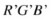directly, three derived components are sent—namely the luma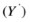and two color difference signals (B 'Y ') and (R' – Y').

While in analog video, these color difference signals are represented by U and V, respectively, in digital video, they are known as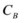and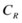components, respectively. In fact, U and V apply to analog video only, but are commonly, albeit inappropriately, used in digital video as well. The term chroma represents the color difference signals themselves; this term should not be confused with chromaticity, which represents the characteristics of the color signals.

In particular, chromaticity refers to an objective measure of the quality of color information only, not accounting for the luminance quality. Chromaticity is characterized by the hue and the saturation. The hue of a color signal is its “redness,” “greenness,” and so on. The hue is measured as degrees in a color wheel from a single hue. The saturation or colorfulness of a color signal is the degree of its difference from gray.

Figure 2-6 depicts the chromaticity diagram for the ITU-R recommendation BT.709 and BT.2020, showing the location of the red, green, blue, and white colors. Owing to the differences shown in this diagram, digital video signal represented in BT.2020 color primaries cannot be directly presented to a display that is designed according to BT.709; a conversion to the appropriate color primaries would be necessary in order to faithfully reproduce the actual colors.

In order to convertsamples to corresponding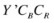samples, in general, the following formulas are used:(Eq. 2-1)

Each of the ITU-R recommendations mentioned previously uses the values of constants K r, K g, and K b, as shown in Table 2-2, although the constant names are not defined as such in the specifications.Table 2-2. Constants of Coefficients to Form Luma and Chroma Components

It is notable that all of these ITU-R recommendations also define a visible range between black and white for the allowed bit depths. For example, according to BT.2020, for 10-bit the luma ranges from 64 to 940; the ranges 0 to 3 and 1020 to 1023 are used for timing reference, while the ranges 4 to 63 and 941 to 1019 provide foot- and headroom, respectively, to accommodate transient black and white signals that may result from overshoots of filters. Similarly, BT.601 and BT.709 define the active range of luma between 16 and 235 for 8-bit video. In the case of 4:2:2 video, values 0 and 255 are reserved for synchorization and are forbidded from the visible picture area. Values 1 to 15 and 236 to 254 provide the relevant foot- and headroom. Table 2-3 gives the signal formats and conversion formula used in these recommendations.

In addition to the signal formats, the recommendations also specify the opto-electronic conversion parameters and the picture characteristics. Table 2-4 shows some of these parameters.

### Chroma Subsampling

As mentioned earlier, the HVS is less sensitive to color information compared to its sensitivity to brightness information. Taking advantage of this fact, technicians developed methods to reduce the chroma information without significant loss in visual quality. Chroma subsampling is a common data-rate reduction technique and is used in both analog and digital video encoding schemes. Besides video, it is also used, for example, in popular single-image coding algorithms, as defined by the Joint Photographic Experts Group (JPEG), a joint committee between the International Standards Organization (ISO) and the ITU-T.

Exploiting the high correlation in color information and the characteristics of the HVS, chroma subsampling reduces the overall data bandwidth. For example, a 2:1 chroma subsampling of a rectangular image in the horizontal direction results in only two-thirds of the bandwidth required for the image with full color resolution. However, such saving in data bandwidth is achieved with little perceptible visual quality loss at normal viewing distances.

#### 4:4:4 to 4:2:0

Typically, images are captured in the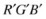color space, and are converted to the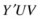color space (or for digital video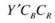C B C R; in the discussion we useand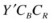C B C R interchangeably for simplicity) using the conversion matrices described earlier. The resulting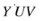image is a full-resolution image with a 4:4:4 sampling ratio of the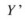, U and V components, respectively. This means that for every four samples of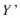(luma), there are four samples of U and four samples of V chroma information present in the image.

The ratios are usually defined for a 4×2 sample region, for which there are four 4×2 luma samples. In the ratio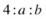, a and b are determined based on the number of chroma samples in the top and bottom row of the 4 × 2 sample region. Accordingly, a 4:4:4 image has full horizontal and vertical chroma resolution, a 4:2:2 image has a half-horizontal and full vertical resolution, and a 4:2:0 image has half resolutions in both horizontal and vertical dimensions.

The 4:2:0 is different from 4:1:1 in that in 4:1:1, one sample is present in each row of the 4 × 2 region, while in 4:2:0, two samples are present in the top row, but none in the bottom row. An example of the common chroma formats (4:4:4, 4:2:2 and 4:2:0) is shown in Figure 2-7.

A subsampling is also known as downsampling, or sampling rate compression. If the input signal is not bandlimited in a certain way, subsampling results in aliasing and information loss, and the operation is not reversible. To avoid aliasing, a low pass filter is used before subsampling in most appplications, thus ensuring the signal to be bandlimited.

The 4:2:0 images are used in most international standards, as this format provides sufficient color resolution for an acceptable perceptual quality, exploiting the high correlation between color components. Therefore, often a camera-captured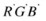image is converted to4:2:0 format for compression and processing. In order to convert a 4:4:4 image to a 4:2:0 image, typically a two-step approach is taken. First, the 4:4:4 image is converted to a 4:2:2 image via filtering and subsampling horizontally; then, the resulting image is converted to a 4:2:0 format via vertical filtering and subsampling. Example filters are shown in Figure 2-8.

The filter coefficients for the Figure 2-8 finite impulse response (FIR) filters are given in Table 2-5. In this example, while the horizontal filter has zero phase difference, the vertical filter has a phase shift of 0.5 sample interval.

### Reduction of Redundancy

Digital video signal contains a lot of similar and correlated information between neighboring pixels and neighboring frames, making it an ideal candidate for compression by removing or reducing the redundancy. We have already discussed chroma subsampling and the fact that very little visual difference is seen because of such subsampling. In that sense, the full resolution of chroma is redundant information, and by doing the subsampling, a reduction in data rate—that is, data compression—is achieved. In addition, there are other forms of redundancy present in a digital video signal.

#### Spatial Redundancy

The digitization process ends up using a large number of bits to represent an image or a video frame. However, the number of bits necessary to represent the information content of a frame may be substantially less, due to redundancy. Redundancy is defined as 1 minus the ratio of the minimum number of bits needed to represent an image to the actual number of bits used to represent it. This typically ranges from 46 percent for images with a lot of spatial details, such as a scene of foliage, to 74 percentFootnote 14 for low-detail images, such as a picture of a face. Compression techniques aim to reduce the number of bits required to represent a frame by removing or reducing the available redundancy.

Spatial redundancy is the consequence of the correlation in horizontal and the vertical spatial dimensions between neighboring pixel values within the same picture or frame of video (also known as intra-picture correlation). Neighboring pixels in a video frame are often very similar to each other, especially when the frame is divided into the luma and the chroma components. A frame can be divided into smaller blocks of pixels to take advantage of such pixel correlations, as the correlation is usually high within a block. In other words, within a small area of the frame, the rate of change in a spatial dimension is usually low. This implies that, in a frequency-domain representation of the video frame, most of the energy is often concentrated in the low-frequency region, and high-frequency edges are relatively rare. Figure 2-9 shows an example of spatial redundancy present in a video frame.

The redundancy present in a frame depends on several parameters. For example, the sampling rate, the number of quantization levels, and the presence of source or sensor noise can all affect the achievable compression. Higher sampling rates, low quantization levels, and low noise mean higher pixel-to-pixel correlation and higher exploitable spatial redundancy.

#### Temporal Redundancy

Temporal redundancy is due to the correlation between different pictures or frames in a video (also known as inter-picture correlation). There is a significant amount of temporal redundancy present in digital videos. A video is frequently shown at a frame rate of more than 15 frames per second (fps) in order for a human observer to perceive a smooth, continuous motion; this requires neighboring frames to be very similar to each other. One such example is shown in Figure 2-10. It may be noted that a reduced frame rate would result in data compression, but that would be at the expense of perceptible flickering artifact.

Thus, a frame can be represented in terms of a neighboring reference frame and the difference information between these frames. Because an independent frame is reconstructed at the receiving end of a transmission system, it is not necessary for a dependent frame to be transmitted. Only the difference information is sufficient for the successful reconstruction of a dependent frame using a prediction from an already received reference frame. Due to temporal redundancy, such difference signals are often quite small. Only the difference signal can be coded and sent to the receiving end, while the receiver can combine the difference signal with the predicted signal already available and obtain a frame of video, thereby achieving very high amount of compression. Figure 2-11 shows an example of how temporal redundancy is exploited.

The difference signal is often motion-compensated to minimize the amount of information in it, making it amenable to a higher compression compared to an uncompensated difference signal. Figure 2-12 shows an example of reduction of information using motion compensation from one video frame to another.

The prediction and reconstruction process is lossless. However, it is easy to understand that the better the prediction, the less information remains in the difference signal, resulting in a higher compression. Therefore, every new generation of international video coding standards has attempted to improve upon the prediction process of the previous generation.

#### Statistical Redundancy

In information theory, redundancy is the number of bits used to transmit a signal minus the number of bits of actual information in the signal, normalized to the number of bits used to transmit the signal. The goal of data compression is to reduce or eliminate unwanted redundancy. Video signals characteristically have various types of redundancies, including spatial and temporal redundancies, as discussed above. In addition, video signals contain statistical redundancy in its digital representation; that is, there are usually extra bits that can be eliminated before transmission.

For example, a region in a binary image (e.g., a fax image or a video frame) can be viewed as a string of 0s and 1s, the 0s representing the white pixels and 1s representing the black pixels. These strings, where the same bit occurs in a series or run of consecutive data elements, can be represented using run-length codes; these codes the address of each string of 1s (or 0s) followed by the length of that string. For example, 1110 0000 0000 0000 0000 0011 can be coded using three codes (1,3), (0,19), and (1,2), representing 3 1s, 19 0s, and 2 1s. Assuming only two symbols, 0 and 1, are present, the string can also be coded using two codes (0,3) and (22,2), representing the length of 1s at locations 0 and 22.

Variations on the run-length are also possible. The idea is this: instead of the original data elements, only the number of consecutive data elements is coded and stored, thereby achieving significant data compression. Run-length coding is a lossless data compression technique and is effectively used in compressing quantized coefficients, which contains runs of 0s and 1s, especially after discarding high-frequency information.

According to Shannon’s source coding theorem, the maximum achievable compression by exploiting statistical redundancy is given as: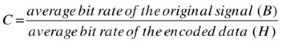Here, H is the entropy of the source signal in bits per symbol. Although this theoretical limit is achievable by designing a coding scheme, such as vector quantization or block coding, for practical video frames—for instance, video frames of size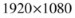pixels with 24 bits per pixel—the codebook size can be prohibitively large.Footnote 15 Therefore, international standards instead often use entropy coding methods to get arbitrarily close to the theoretical limit.

### Entropy Coding

Consider a set of quantized coefficients that can be represented using B bits per pixel. If the quantized coefficients are not uniformly distributed, then their entropy will be less than B bits per pixel. Now, consider a block of M pixels. Given that each bit can be one of two values, we have a total number of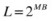different pixel blocks.

For a given set of data, let us assign the probability of a particular block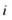occurring as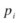, where. Entropy coding is a lossless coding scheme, where the goal is to encode this pixel block using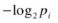bits, so that the average bit rate is equal to the entropy of the M pixel block: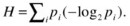. This gives a variable length code for each block of M pixels, with smaller code lengths assigned to highly probable pixel blocks. In most video-coding algorithms, quantized coefficients are usually run-length coded, while the resulting data undergo entropy coding for further reduction of statistical redundancy.

For a given block size, a technique called Huffman coding is the most efficient and popular variable-length encoding method, which asymptotically approaches Shannon’s limit of maximum achievable compression. Other notable and popular entropy coding techniques are arithmetic coding and Golomb-Rice coding.

Golomb-Rice coding is especially useful when the approximate entropy characteristics are known—for example, when small values occur more frequently than large values in the input stream. Using sample-to-sample prediction, the Golomb-Rice coding scheme produces output rates within 0.25 bits per pixel of the one-dimensional difference entropy for entropy values ranging from 0 to 8 bits per pixel, without needing to store any code words. Golomb-Rice coding is essentially an optimal run-length code. To compare, we discuss now the Huffman coding and the arithmetic coding.

#### Huffman Coding

Huffman coding is the most popular lossless entropy coding algorithm; it was developed by David Huffman in 1952. It uses a variable-length code table to encode a source symbol, while the table is derived based on the estimated probability of occurrence for each possible value of the source symbol. Huffman coding represents each source symbol in such a way that the most frequent source symbol is assigned the shortest code and the least frequent source symbol is assigned the longest code. It results in a prefix code, so that a bit string representing a source symbol is never a prefix of the bit string representing another source symbol, thereby making it uniquely decodable.

To understand how Huffman coding works, let us consider a set of four source symbols {a 0, a 1, a 2, a 3} with probabilities {0.47, 0.29, 0.23, 0.01}, respectively. First, a binary tree is generated from left to right, taking the two least probable symbols and combining them into a new equivalent symbol with a probability equal to the sum of the probablities of the two symbols. In our example, therefore, we take a 2 and a 3 and form a new symbol b 2 with a probability 0.23 + 0.01 = 0.24. The process is repeated until there is only one symbol left.

The binary tree is then traversed backwards, from right to left, and codes are assigned to different branches. In this example, codeword 0 (one bit) is assigned to symbol a 0, as this is the most probable symbol in the source alphabet, leaving codeword 1 for c 1. This codeword is the prefix for all its branches, ensuring unique decodeability. At the next branch level, codeword 10 (two bits) is assigned to the next probable symbol a 1, while 11 goes to b 2 and as a prefix to its branches. Thus, a 2 and a 3 receive codewords 110 and 111 (three bits each), respectively. Figure 2-13 shows the process and the final Huffman codes.

While these four symbols could have been assigned fixed length codes of 00, 01, 10, and 11 using two bits per symbol, given that the probability distribution is non-uniform and the entropy of these symbols is only 1.584 bits per symbol, there is room for improvement. If these codes are used, 1.77 bits per symbol will be needed instead of two bits per symbol. Although this is still 0.186 bits per symbol apart from the theoretical minimum of 1.584 bits per symbol, it still provides approximately 12 percent compression compared to fixed-length code. In general, the larger the difference in probabilities between the most and the least probable symbols, the larger the coding gain Huffman coding would provide. Huffman coding is optimal when the probability of each input symbol is the inverse of a power of 2.

#### Arithmetic Coding

Arithmetic coding is a lossless entropy coding technique. Arithmetic coding differs from Huffman coding in that, rather than separating the input into component symbols and replacing each with a code, arithmetic coding encodes the entire message into a single fractional number between 0.0 and 1.0. When the probability distribution is unknown, not independent and not identically distributed, arithmetic coding may offer better compression capability than Huffman coding, as it can combine an arbitrary number of symbols for more efficient coding and is usually adaptable to the actual input statistics. It is also useful when the probability of one of the events is much larger than ½. Arithmetic coding gives optimal compression, but it is often complex and may require dedicated hardware engines for fast and practical execution.

In order to describe how arithmetic codingFootnote 16 works, let us consider an example of three events (e.g., three letters in a text): the first event is either a 1 or b 1, the second is either a 2 or b 2, and the third is either a 3 or b 3. For simplicity, we choose between only two events at each step, although the algorithm works for multi-events as well. Let the input text be b 1 a 2 b 3, with probabilities as given in Figure 2-14.

## Compression Techniques: Cost-benefit Analysis

In this section we discuss several commonly used video-compression techniques and analyze their merits and demerits in the context of typical usages.

### Transform Coding Techniques

As mentioned earlier, pixels in a block are similar to each other and have spatial redundancy. But a block of pixel data does not have much statistical redundancy and is not readily suitable for variable-length coding. The decorrelated representation in the transform domain has more statistical redundancy and is more amenable to compression using variable-length codes.

In transform coding, typically a video frame of size N×M is subdivided into smaller n×n blocks, and a reversible linear transform is applied on these blocks. The transform usually has a set of complete orthonormal discrete-basis functions, while its goal is to decorrelate the original signal and to redistribute the signal energy among a small set of transform coefficients. Thus, many coefficients with low signal energy can be discarded through the quantization process prior to coding the remaining few coefficients. A block diagram of transform coding is shown in Figure 2-15.

#### Discrete Cosine Transform

A discrete cosine transform (DCT) expresses a finite sequence of discrete data points in terms of a sum of cosine functions with different frequencies and amplitudes. The DCT is a linear, invertible, lossless transform that can very effectively decorrelate the redundancy present in a block of pixels. In fact, the DCT is the most efficient, practical transform available for this purpose and it approaches the theoretically optimum Karhunen-Loève transform (KLT), as very few cosine functions are needed to approximate a typical signal. For this reason, the DCT is widely used in video and audio compression techniques.

There are four representations of the DCT, of which DCT-IIFootnote 17 is the most common form: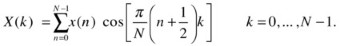Here, X k is the transformed DCT coefficient, and x n is the input signal. This one-dimensional DCT can be separately used vertically and horizontally, one after the other, to obtain a two-dimensional DCT. For image and video compression, the DCT is most popularly performed on 8×8 blocks of pixels. The 8×8 two-dimensional DCT can be expressed as follows: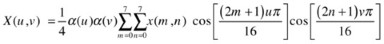Here, u and v are the horizontal and vertical spatial frequencies, 0 ≤ u, v < 8; α(k) is a normalizing factor equal to 1/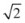for k = 0, and equal to 1 otherwise; x(m,n) is the pixel value at spatial location (m,n); and X(u,v) is the DCT coefficient at frequency coordinates (u,v).

The DCT converts an 8×8 block of input values to a linear combination of the 64 two-dimensional DCT basis functions, which are represented in 64 different patterns, as shown in Figure 2-16.

Although the transform is lossless, owing to limitations in arithmetic precision of a computing system, it may introduce inaccuracies so that the same, exact input may not be obtained upon an inverse operation. In order to handle such inaccuracies, standard committees often take measures such as defining the IEEE standard 1180, which is described later in this chapter.

A signal flow diagram of an eight-point DCT (and inverse DCT) is shown in Figure 2-17, representing a one-dimensional DCT, where the input data set is (u 0, . . . , u 7), the output data set is (v 0, . . . , v 7), and (f 0, . . . , f 7) are the cosine function-based multiplication factors for the intermediate results. There are many fast algorithms and implementations of the DCT available in the literature, as nearly all international standards adopt the DCT as the transform of choice to reduce spatial redundancy.

The DCT can easily be implemented using hardware or software. An optimized software implementation can take advantage of single instruction multiple data (SIMD) parallel constructs available in multimedia instruction sets such as MMX or SSE.Footnote 18 Furthermore, there are dedicated hardware engines available in Intel integrated graphics processor based codec solutions.

An example of a block of pixels and its DCT-transformed coefficients is depicted in Figure 2-18.

##### Quantization

As the DCT is characteristically lossless, it does not provide compression by itself; it merely decorrelates the input data. However, the DCT is usually followed by a quantization process, which truncates the high-frequency information of the transformed data block, exploiting the spatial redundancy present in frames of video.

A quantizer is a staircase function that maps a continuous input signal or a discrete input signal with many values, into a smaller, finite number of output levels. If x is a real scalar random variable with p(x) being its probability density function, a quantizer maps x into a discrete variable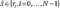, where each level r i is known as a reconstruction level. The values of x that map to a particular x* are defined by a set of decision levels {d i, i = 0, . . . , N−1}. According to the quantization rule, if x lies in the interval (d i, d i+1], it is mapped—that is, quantized to r i—which also lies in the same interval. Quantizers are designed to optimize the r i and d i for a given p(x) and a given optimization criterion.

Figure 2-19 shows an example eight-level nonlinear quantizer. In this example, any value of x between (-255, 16] is mapped to -20, similarly any value between (-16, -8] is mapped to -11, any value between (-8, -4] is mapped to -6, and so on. This quantization process results in only eight nonlinear reconstruction levels for any input value between (-255, 255).

After quantization, an 8×8 transform data block typically reduces from 64 coefficients to from 5 to 10 coefficients, and approximately 6- to 12-fold data compression is usually achieved. However, note that quantization is a lossy process where the discarded high-frequency information cannot be regained upon performing the inverse operation. Although the high-frequency information is frequently negligible, that is not always the case. Thus, the transform and quantization process usually introduces a quality loss, which is commonly known as the quantization noise. All international standards define the transform and quantization process in detail and require conformance to the defined process.

In the case of a two-dimensional signal, such as an image or a video frame where quantization is usually performed on blocks of pixels, contouring effect is produced at the block boundaries because the blocks are transformed and quantized independently; as a result, the block boundaries become visible. This is commonly known as the blocking or blocky artifact. Although a coarser quantization level would yield a greater data compression, it is worthwhile to note that the coarser the quantization level for a signal, the more blocking artifact will be introduced.

The Walsh-Hadamard transform (WHT) is a linear, orthogonal, and symmetric transform that usually operate on 2m real numbers. It has only modest decorrelation capability, but it is a popular transform owing to its simplicity. The WHT basis functions consist of values of either +1 or -1, and can be obtained from the rows of orthonormal Hadamard matrices. Orthonormal Hadamard matrices can be constructed recursively from the smallest 2 × 2 matrix of the same kind, which is a size 2 discrete Fourier transform (DFT), as follows: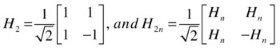There are fast algorithms available for computation of the Hadamard transform, making it suitable for many applications, including data compression, signal processing, and data encryption algorithms. In video-compression algorithms, it is typically used in the form of sum of absolute transform differences (SATD), which is a video-quality metric used to determine if a block of pixel matches another block of pixel.

There are other less frequently used transforms found in various video-compression schemes. Notable among them is the discrete wavelet transform (DWT), the simplest form of which is called the Haar transform (HT). The HT is an invertible, linear transform based on the Haar matrix. It can be thought of as a sampling process in which rows of the Haar matrix act as samples of finer and finer resolution. It provides a simple approach to analyzing the local aspects of a signal, as opposed to non-localized WHT, and is very effective in algoithms such as subband coding. An example of a 4×4 Haar matrix is this: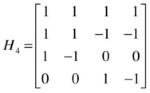### Predictive Coding Techniques

Prediction is an important coding technique. In the receiving end of a transmission system, if the decoder can somehow predict the signal, even with errors, it can reconstruct an approximate version of the input signal. However, if the error is known or transmitted to the decoder, the reconstruction will be a more faithful replica of the original signal. Predictive coding takes advantage of this principle. Predictive coding can be lossy or lossless. Here are some predictive techniques.

#### Lossless Predictive Coding

By exploiting the spatial redundancy, a pixel can be predicted from its neighbor. As the difference between the neighbors is usually small, it is more efficient to encode the difference rather than the actual pixel. This approach is called differential pulse code modulation (DPCM) technique. In DPCM, the most probable estimates are stored, and the difference between the actual pixel x and its most likely prediction x' is formed. This difference, e = xx', is called the error signal, which is typically entropy coded using variable-length codes.

To get a better estimate, the prediction can be formed as a linear combination of a few previous pixels. As the decoder already decodes the previous pixels, it can use these to predict the current pixel to obtain x', and upon receiving the error signal e, the decoder can perform e + x' to obtain the true pixel value. Figure 2-20 shows the concept.

In the Figure 2-20 example, the current pixel X can be predicted from a linear combination of the previously decoded pixels; for example, depending on the correlation, X = 0.75A – 0.25B + 0.5C can be a good predicted value for X. The error image usually has a reduced variance and much less spatial correlation compared to the original image. Therefore, in DPCM, the error image is coded using a variable-length code such as the Huffman code or the arithmetic code. This approach yields the desired lossless compression.

There are some applications—for instance, in medical imaging—that benefit from combining lossless and lossy predictions, mainly to achieve a shorter transmission time. However, these applications may tolerate only small quality degradation and very high quality reconstructions are expected. In such cases, a low bit-rate version of the image is first constructed by using an efficient lossy compression algorithm. Then, a residual image is generated by taking the difference between the lossy version and the original image, which is followed by a lossless coding of the residual image.

#### Lossy Predictive Coding

In order to accommodate a reduced bit rate, some visual quality loss is allowed in lossy coding, while greater compression may be achieved by allowing more quality degradation. Figure 2-21 shows the general concept of lossy coding, where the original image is decomposed and/or transformed to frequency domain, the frequency-domain information is quantized, and the remaining information is coded using entropy coding.

The decomposition and transformation reduce the dynamic range of the signal and also decorrelate the signal, resulting in a form that can be coded more efficiently. This step is usually reversible and lossless. However, in the next step, quantization is performed, which introduces information loss and consequently quality degradation but achieves compression as well. The entropy coding is again a lossless process, but it provides some compression by exploiting statistical redundancy.

##### Lossy DPCM

In predictive coding, as mentioned earlier in connection with lossless DPCM, a prediction or estimate is formed based on a reference, then an error signal is generated and coded. However, DPCM schemes can be used in lossy coding as well, resulting in lossy predictive coding.

The reference used for the prediction can be the original signal; however, the decoder at the receiving end of the transmission channel would only have the partially reconstructed signal based on the bits received so far. Note that the received signal is reconstructed from a quantized version of the signal and contains quantization noise. Therefore, typically there is a difference between the reconstructed and the original signal.

In order to ensure identical prediction at both ends of the transmission channel, the encoder also needs to form its predictions based on the reconstructed values. To achieve this, the quantizer is included in the prediction loop, as shown in Figure 2-22, which essentially incorporates the decoder within the encoding structure.

##### Temporal Prediction

In addition to the prediction from a neighboring pixel exploiting spatial redundancy, prediction may be formed from neighboring frames, exploiting temporal redundancy. Since neighboring frames are similar except for the small movement of objects from one frame to another, the difference signal can be captured and the residual frame can be compensated for the motion.

When the frame is divided in blocks, each block may move to a different location in the next frame. So motion vectors are usually defined for each block to indicate the amount of movement in horizontal and vertical dimensions. The motion vectors are integers and expressed as mv(x,y); however, motion vectors from a subsampled residual frame can be combined with those from the original resolution of the residual frame such that the subsampled motion vectors are expressed as fractions. Figure 2-23 illustrates this concept, where the final motion vector is 12.5 pixels away horizontally in the current frame from the original location (0, 0) in the reference frame; using a half-pixel (or half-pel) precision of motion vectors.

In order to perform motion compensation, motion vectors are determined using a process called motion estimation, which is typically done for a 16×16 pixel block or picture partitions of other shapes. The motion-estimation process defines a search window in the reference frame where a search is performed for the best maching block relative to the current block in the current frame. The search window is usually formed around the co-located (0, 0) position, which has the same horizontal and vertical co-ordinates in the reference frame compared to the current block in the current frame. However, in some algorithms, the search windows may be formed around a predicted motion vector candidate as well. A matching criterion, typically a distortion metric, is defined to determine the best match.

This method of block-matching motion estimation is different from a pel-recursive motion estimation, which involves matching all the pixels of the frame in a recursive manner. Figure 2-24 illustrates an example of a block-matching motion estimation.

There are a large number of motion-estimation algorithms available in the literature. Block-matching techniques attempt to minimize the distortion metric and try to find a global minimum distance between the two blocks within the search area. Typical distortion metrics are mean absolute difference (MAD), sum of absolute difference (SAD), and sum of absolute transform difference (SATD) involving Haar transform, having different computational complexities and matching capabilities. The motion estimation is a computationally intensive process—so much so that the encoding speed is largely determined by this process. Therefore, the choice of the distortion metric is important in lossy predictive video coding.

There are additional popular coding algorithms, including vector quantization and subband coding. These algorithms are also well known owing to their individual, special characteristics.

#### Vector Quantization

In vector quantization (VQ), a frame of video is decomposed into an n-dimensional vector. For example, the Y C B C R components may form a three-dimensional vector, or each column of a frame may be used as elements of the vectors forming a w-dimensional vector, where w is the width of the frame. Each image vector X is compared to several codevectors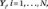which are taken from a previously generated codebook.

Based on a minimum distortion criterion, such as the mean square error (MSE), the comparison results in a best match between X and Y k, the k th codevector. The index k is transmitted using log2 N bits. At the receiving end, a copy of the codebook is already available, where the decoder simply looks up the index k from the codebook to reproduce Y k. Figure 2-25 shows the VQ block diagram.

Compression is achieved because a codebook with relatively few codevectors is used compared to the number of possible codevectors. Although theoretically VQ can achieve compression efficiencyFootnote 19 close to the rate-distortion bound, in practice an unreasonably large value of n is needed. However, with modest dimensions, sensible compression efficiency can still be achieved, using smart training algorithms. A detailed discussion of VQ can be found in Rabbani and Jones.Footnote 20

#### Subband Coding

In subband coding (SBC) technique, an image is filtered to create a set of images called subbands, each with limited spatial frequencies. As each subband has reduced bandwidth, a subsampled version of the original image is used for each subband. The process of filtering and subsampling is known as the analysis stage. The subbands are then encoded using one or more encoders, possibly using different encode parameters. This allows the coding error to be distributed among different subbands so that a visually optimal reconstruction can be achieved by performing a corresponding upsampling, filtering, and subsequent combining of the subbands. This manner of reconstruction is known as the synthesis stage.

Subband decomposition by itself does not provide any compression. However, subbands can be coded more efficiently compared to the original image, thereby providing an overall compression benefit. Figure 2-26 shows a block diagram of the scheme. Many coding techniques may be used for coding of different subbands, including DWT, Haar transform, DPCM, and VQ. An elaborate discussion on SBC can be found in Rabbani and Jones.Footnote 21

### Rate-Distortion Theory

The source entropy defines the minimum number of bits necessary to encode an image or video frame. However, this only applies to lossless encoding. In practice, owing to the characteristics of the human visual system, some irreversible visual quality loss can be tolerated. The extent of loss can be controlled by adjusting encode parameters such as quantization levels.

In order to determine an acceptable amount of visual degradation for a given number of bits, a branch of information theory called the rate-distortion theory was developed. The theory establishes theoretical bounds on compression efficiency for lossy data compression, according to a fidelity criterion, by defining a rate-distortion function R(D) for various distortion measures and source models. The function has the following properties:

• For a given distortion D, a coding scheme exists for which a rate R(D) is obtained with a distortion D.

• For any coding scheme, R(D) represents the minimum rate for a given distortion D.

• R(D) is a convex cup ∪, and continuous function of D.

Figure 2-27 shows a typical rate-distortion function. For distortion-free or visually lossless compression, the minimum rate required is the value of R at D = 0, which may be equal to or less than the source entropy, depending on the distortion measure.

The R(D) bound depends on the source model and distortion measures. Usually encoders can achieve compression efficiency closer to R(D) at the expense of higher complexity; a better encoder uses a lower rate and tolerates a lower distortion, but may have higher complexity compared to another encoder. To determine compression efficiency relative to R(D), a two-dimensional Gauss-Markov source image model with unity correlation coefficient is often used as a reference. However, for natural image and video, finding good source model and suitable distortion criteria that correlate well with the human visual system is a topic of active research.

#### Lossy Compression Aspects

There are several factors that influence and distinguish compression algorithms. These factors should be carefully considered while tuning or choosing a compression algorithm for a particular usage model. Among these factors are:

• Sensitivity to input frame types: Compression algorithms may have different compression efficiencies based on input frame characteristics, such as dynamic range, camera noise, amount of pixel to pixel correlation, resolution, and so on.

• Target bit rate: Owing to limited bandwidth availability, some applications may need to adhere to a certain bit rate, but would sacrifice visual quality if needed. Compression algorithms usually have different sweet spots in the rate-distortion curve, and target bit rates outside its sweet spots would result in poor visual quality. Some algorithms may not be able to operate below a certain bit rate; for example, the AVC algorithm for HD resolution may need to use more than 1.5 Mbps for any meaningful visual quality), regardless of the spatio-temporal complexity. This bit rate corresponds to approximately 500 times compression for a 1920 × 1080 resolution at 30 fps video.

• Constant bit rate vs. constant quality: Some algorithms are more suitable for transmission without buffering, as they operate with a constant bit rate. However, they may need to maintain the bit rate at the expense of visual quality for complex scenes. As video complexity varies from scene to scene, the constant bit rate requirement will result in a variable reconstruction quality. On the other hand, some algorithms maintain a somewhat constant quality throughout the video by allowing a fixed amout of distortion, or by adjusting levels of quantization based on the scene complexity. In doing so, however, they end up with a variable bit rate, which may require adequate buffering for transmission.

• Encoder-decoder asymmetry: Some algorithms, such as vector quantization schemes, use a very complex encoder, while the decoder is implemented with a simple table look-up. Other schemes, such as the MPEG algorithms, need a higher decoder complexity compared to vector quantization, but simplify the encoder. However, MPEG encoders are typically more complex than the decoders, as MPEG encoders also contain complete decoders within them. Depending on the end-user platform, certain schemes may be more suitable than others for a particular application.

• Complexity and implementation issues: The computational complexity, memory requirements, and openness to parallel processing are major differentiating factors for hardware or software implementation of compression algorithms. While software-based implementations are more flexible to parameter tuning for highest achievable quality and are amenable to future changes, hardware implementations are usually faster and power-optimized. Appropriate tradeoff is called for depending on end-ser platform and the usage model.

• Error resilience: Compressed data is usually vulnerable to channel errors, but the degree of susceptibility varies from one algorithm to another. DCT-based algorithms may lose one or more blocks owing to channel errors, while a simple DPCM algorithm with variable-length codes may be exposed to the loss of an entire frame. Error-correcting codes can compensate for certain errors at the cost of complexity, but often this is cost-prohitive or does not work well in case of burst errors.

• Artifacts: Lossy compression algorithms typically produce various artifacts. The type of artifacts and its severity may vary from one algorithm to another, even at the same bit rate. Some artifacts, such as visible block boundaries, jagged edges, ringing artifacts around objects, and the like, may be visually more objectionable than random noise or a softer image. Also, the artifacts are dependent on nature of the content and the viewing condition.

• Effect of multi-generational coding: Applications such as video editing may need multiple generations of coding and decoding, where a decoded output is used as the input to the encoder again. The output from the encoder is a second-generation compressed output. Some applications support multiple such generations of compression. Some compression algorithms are not suitable for multi-generational schemes, and often result in poor quality after the second generation of encoding the same frame.

• System compatibility: Not all standards are available on all systems. Although one of the goals of standardization is to obtain use of common format across the industry, some vendors may emphasize one compression algorithm over another. Although standardization yields commonly acknowledges formats such as AVC and HEVC, vendors may choose to promote similar algorithm such as VC-1, VP8, or VP9. Overall, this is a larger issue encompassing definitions of technologies such as Blu-ray vs. HD-DVD. However, compatibility with the targeted eco-system is a factor worthy of consideration when choosing a compression solution.

## Summary

We first discussed typical compression requirements for video transmission with various networks. This was followed by a discussion of how the characteristics of the human visual system provide compression opportunities for videos. Then, aiming to familiarize the reader with various video compression methods and concepts, in particular the most popular technologies, we presented various ways to perform compression of digital video. We explained a few technical terms and concepts related to video compression. Then we presented the various compression techniques targeted to reducing spatial, temporal, and statistical redundancy that are available in digital video. We briefly described important video coding techniques, such as transform coding, predictive coding, vector quantization, and subband coding. These techniques are commonly employed in presently available video-compression schemes. We then introduced the rate-distortion curve as the compression efficiency metric, and as a way of comparing two encoding solutions. Finally, we presented the various factors that influence the compression algorithms, the understanding of which will facilitate choosing a compression algorithm.